Cats2D Multiphysics > Research Topics > Unstable thermal convection in VB growth

Unstable thermal convection in vertical Bridgman growth

I have collated a series of entries from my developments page into this article that analyzes the stability of a vertical Bridgman system in which a Hopf bifurcation causes onset of an oscillatory buoyancy-driven flow.

Conditions of simulation

These calculations were done using the parabolic "roll-off" type temperature profile discussed in my article on the rotating baffle vertical Bridgman method. On the left below I show two examples of this profile. A steady solution computed using the gently curved red profile profile is shown to the right.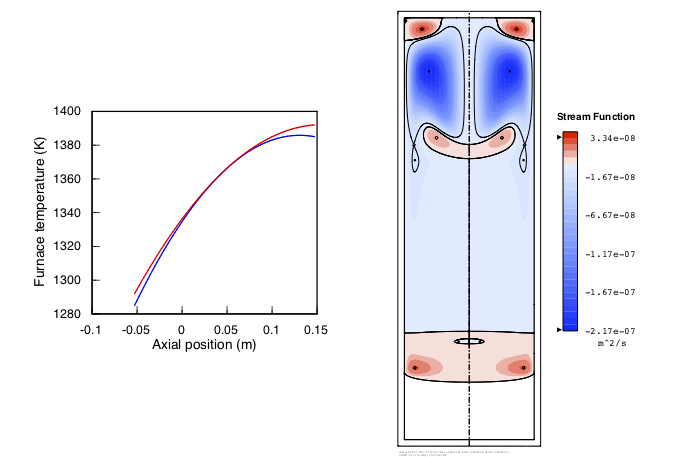No steady state exists for the more sharply curved blue profile, which has a destabilizing gradient near the top of the melt. Time-dependent buoyant flow is shown below, played at normal speed (left) and 1/10th speed (right). The flow is nearly periodic with a 12 second interval. In the regions shaded red, fluid rises near the center and sinks near the walls. In the regions shaded blue, fluid sinks near the center and rises nears the walls.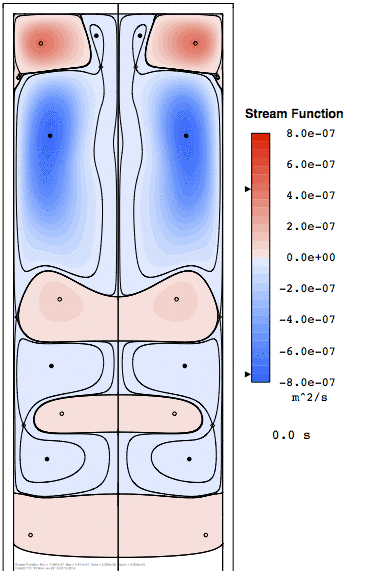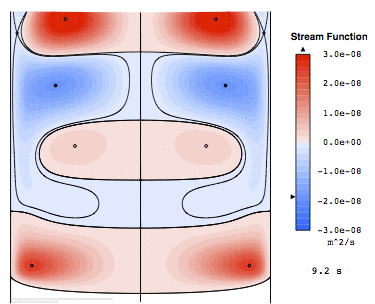The flow evolves slowly over time as the growth interface moves upward, but only 3 microns over one cycle, so it takes many cycles to notice any evolution in the flow or change in the interval.

Detecting instability by time integration of batch growth

Below I show streamline plots after 10, 60, and 120 hours of simulated growth started from this solution. The thermophysical properties are those of cadmium telluride in a quartz ampoule of 5 cm diameter.The results shown here were computed using a fixed time step of 60 seconds. The integration converged at every time step without any obvious growth of discretization error. I had every reason to believe these were good calculations. But here at Cats2D we sleep with one eye open, if we sleep at all, so I tried repeating the integration with a fixed time step of 30 seconds. After just a few minutes of simulated growth the computation failed to converge when the quasi-steady flow began exhibiting time-dependent behavior on a time scale much shorter than the time step. It appears that a large time step allows the integration to “step over” some fast dynamics that happen in the early part of growth. I write more about this here.

To explore this further I repeated the integration using variable step size with error control. The plot below shows how step size adjusts to capture the flow dynamics. We learn that step size must be reduced to around 2 seconds to capture the time-dependent flow without losing convergence. But we also need to be careful about capping step size. Initializing step size to 1 second, but allowing it to grow without bound, results in the upper curve in the figure. Step size grows large enough fast enough to step over the early dynamics. Capping it at 60 seconds or less, shown by the two lower curves, is necessary to avoid doing so.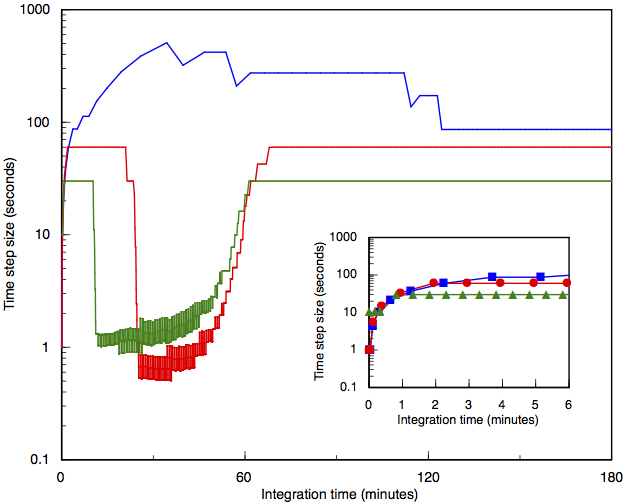It appears the initial condition is unstable to perturbations even though it is a converged steady state. Within a few hundred time steps the flow evolves into a limit cycle with a period of 53 seconds, the topology of which is animated below.These observations indicate that a Hopf bifurcation has occurred, which is characterized by a complex conjugate pair of eigenvalues with positive real part in the solution space. This oscillation occurs when the temperature gradient is destabilizing near the top of the ampoule. Formally this oscillation is known as a limit cycle, and it occurs when an eigenvalue of the system has positive real part and non-zero imaginary part.

Analyzing the limit cycle

To study this situation further, I wheeled out our newly restored eigensolver and ran some tests. We are solving the generalized eigenproblem, and the mass matrix is indeterminate because of incompressibility, so we need to use a shift-and-invert strategy to find any useful eigenvalues. The shift is vital to the outcome of the method because it determines which eigenvalues are emphasized in the low order projection of the system onto the Arnoldi vectors. I've put together some notes on linear stability analysis and the eigenproblem to help the non-expert understand these results better.

I started by computing ten leading eigenvalues using zero shift, which are shown below left. The real part is the growth rate of the mode, and the imaginary part is its frequency. All modes have negative real part and zero imaginary part, indicating non-oscillating modes that decay with time. On the right below, results obtained using a complex shift of (0.1, 0.1i) are shown. This shift finds a complex mode with positive growth rate and frequency near 0.1 radian/s. The conjugate of this mode is found by using the conjugate shift (0.1, -0.1i). The x-axis has been expanded by a factor of sixty to show the unstable complex pair. Consequently all the decaying real modes are collapsed under a single symbol close to the origin in this plot.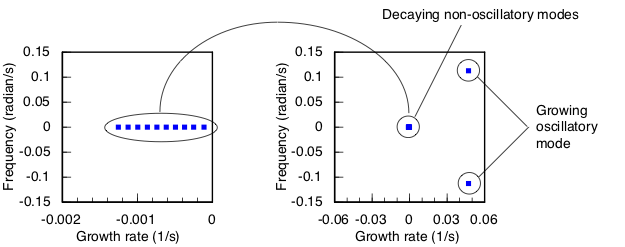For these results I instructed ARPACK to seek eigenvalues with the largest real part. Strictly speaking this means largest real part less than the real component of the shift. Used this way ARPACK will not detect this complex mode unless the real part of the shift is larger than the real part of the eigenvalue. The imaginary part of the shift must also be close to the imaginary part of the eigenvalue. Put another way, we need to hunt for these things if we want to find them. More on this later. For now let's consider what these results are telling us about the system.

If we consider decay time and oscillation period in seconds, essentially the inverse of the quantities plotted here, the slowest decaying real mode has a decay time of 8957 seconds (about 2.5 hours), whereas the unstable complex mode has a growth time of 21.2 seconds and a period of 55.7 seconds. This period is nearly matches the 53 second limit cycle in the animation, which was obtained by direct time integration of the nonlinear equations. Of course the mode does not grow indefinitely; nonlinear effects stabilize its amplitude. I am satisfied the unstable complex eigenmode identified in this linear analysis explains the temporal behavior exhibited by the nonlinear equations.

The slowest decaying real mode is associated with latent heat generated by motion of the growth interface. We can remove this mode by arbitrarily suppressing latent heat generation. The eigenvalues computed using zero shift are shown below left. The effect of this change is to reduce the decay time of the slowest decaying real mode from 8957 seconds to 68 seconds, which is the time scale of thermal transport. Changing the shift to (0.1, ±0.1i) finds the same unstable mode found above, unaffected by suppressing the latent heat.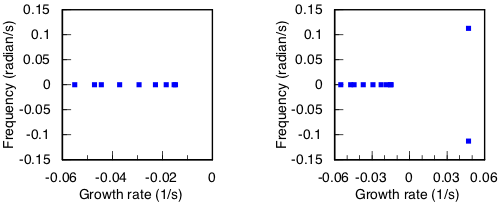We can also arbitrarily knock out contributions to the mass matrix, for example those due to the flow equations. In physical terms this implies the flow is held constant while the temperature field is perturbed. The unstable complex mode vanishes when we do this. If we knock out the energy contributions instead, leaving only the flow contributions, the unstable complex mode remains, though its period is shifted to 35 seconds. From this I conclude the instability is primarily hydrodynamic in nature, but strongly modulated by energy transport.

Next let's consider the behavior of the system when the equations are integrated holding the ampoule stationary to represent a true steady state. Below left shows what happens when integration starts from an unperturbed initial state. There is no change in the solution over the duration of the integration, which was much longer than shown in the plot. It might take a very long time for the instability to grow from numerical noise. Below right shows what happens when the initial state is perturbed by a multiple of the leading eigenmode. The solution trajectory immediately begins oscillating at the period of the mode.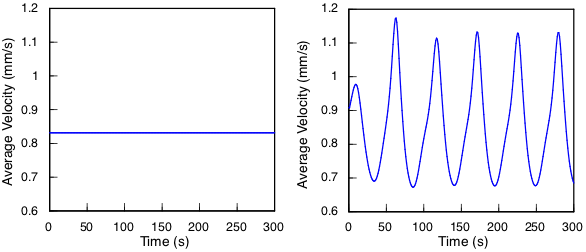Below left shows what happens when integration starts from an unperturbed initial state with the ampoule translating at 1 mm/hour. The simulation is now inherently time-dependent, essentially a batch process, but interface motion is slow enough to expect quasi-steady flow at each time step. Nevertheless, the slight deviation from a true steady state is enough to seed the instability quickly. Below right shows that perturbing the initial state by the leading eigenmode sets off the instability immediately.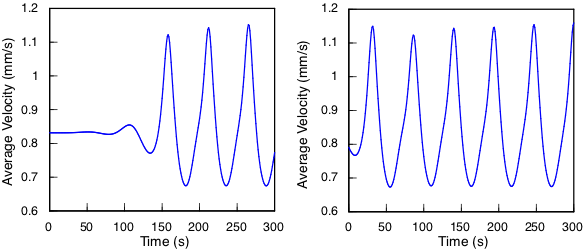The period is unaffected by interface motion over the five minutes of time integration plotted here, but over a longer period of time the ampoule moves into a more stable thermal environment in the lower part of the furnace, allowing the solution to stabilize. The plot of time step size shown in my "Breaking bad" entry below indicates that this happens after about an hour of growth. I've plotted the amplitude of the velocity versus time below to show how the oscillatory mode fades away after which the system exhibits quasi-steady behavior.These integrations were performed using the trapezoid rule with a step size of 0.25 seconds. Here are some additional calculations I've done to ensure this step size is small enough to accurately capture the period and amplitude of the limit cycle.

When the flow stabilizes we can test whether the leading mode has crossed the imaginary axis back into the left half plane. The growth rate and period plotted below predict that the solution stabilizes after about 56 minutes, with a period of 43 seconds. The actual period obtained from the time integration, shown below right by the open circles, is 41.5 seconds at the time of stabilization. Strictly speaking the linear analysis is not valid when applied to a non-stationary solution, but the oscillation is small at 56 minutes, and has nearly vanished at 60 minutes, where the predicted period agrees nearly perfectly with the measured period.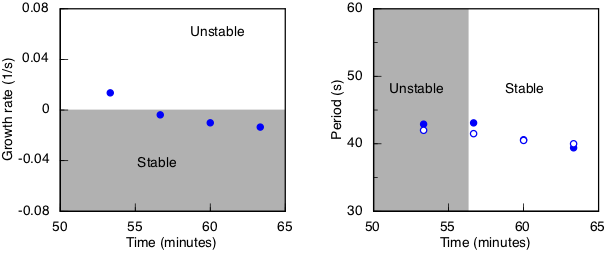A curvature of 2300 K/m2 in the parabolic temperature profile was used in these studies. We can adjust the curvature to find the point at which the initial steady state becomes unstable. The plots below show how the growth rate and period of the leading eigenmode vary as the curvature is increased from 2260 to 2330. The leading eigenmode crosses the imaginary axis at curvature equal to 2283, past which its growth rate is positive. Its period is 43.2 seconds at the crossing, rises to nearly 60 seconds, then falls slightly before solution convergence is lost entirely at curvature equal to 2332.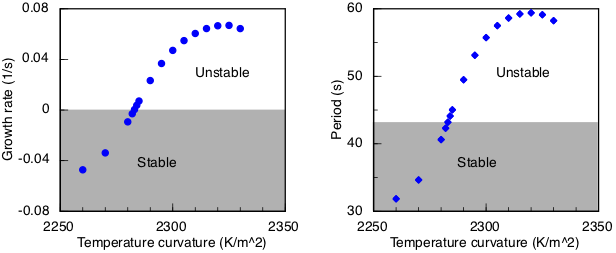Beyond a curvature of 2332 only time dependent solutions exist. The animation shown at the top of this page is a strongly time dependent flow that I computed using a more sharply curved blue profile with curvature equal to 3000. Because no steady state exists for this profile, it was necessary to integrate the equations starting from a quiescent flow. Within a few minutes of simulated time a quasi-periodic flow emerges, but with much shorter period than here, around 12 seconds.

If we want to avoid a time dependent flow we should avoid a destabilizing thermal gradient anywhere in the melt, but what this means quantitatively depends strongly on the size of the system and the thermophysical properties of the material grown in it. The good news is we can model it using Cats2D.

Exploring the eigenspace

Linear stability analysis confirms the occurrence of a Hopf bifurcation leading to a limit cycle. Finding the leading eigenmode was not particularly difficult, but only because I already knew it was there, and knew its frequency too. Thus I was able to quickly find a suitable shift far enough to the right of the imaginary axis to locate the desired eigenmode. Looking blindly would have been more difficult, and I might not have realized to look at all, had I not already stumbled onto the limit cycle by direct time integration of the nonlinear equations.

Generally it is impossible to compute the complete spectrum of eigenvalues for the large matrices we ordinarily use in CFD. In principle there are as many eigenvalues as degrees of freedom in the discretization, so we are computing only a small fraction of a percent of them. Thus it is vital to search in the right places by adjusting the shift thoughtfully.

Let's start striking the piñata. Eigenvalues found using four different shifts are shown in the diagram below. The shift is shown by the open symbol of a type, and the eigenvalues found using that shift are shown by the filled symbols of the same type. What we find depends entirely on where we look.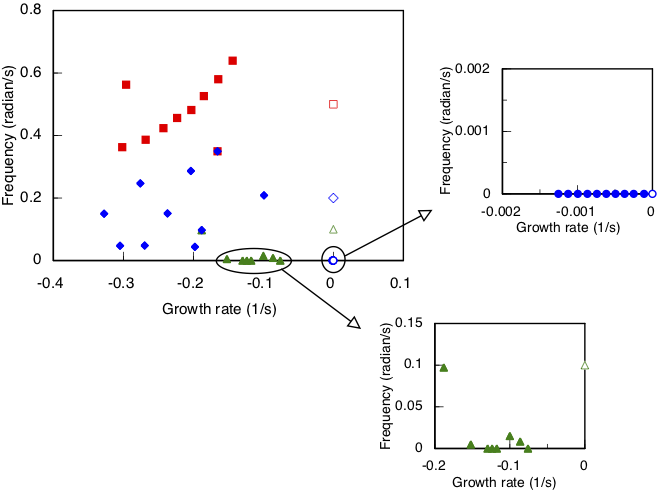The blue circles are found using zero shift. These are clustered near the origin, but we aren't particularly interested in them. They are slow decaying non-oscillatory modes associated with latent heat released at the growth interface and are not the cause of any instabilities in this system. Even though they are close to the imaginary axis, they are not a threat to cross it as long as the temperature gradient at the growth interface remains large enough to maintain morphological stability. The decay time of these modes ranges from about 10 minutes to 2 hours.

The green triangles are found using a shift on the imaginary axis at 0.1i. This shift mostly picks up modes with a decay time around 10 seconds, a few of which oscillate slowly with periods of hundreds of seconds. These modes are associated with thermal transport. The blue diamonds and orange squares are found using higher frequency shifts of 0.2i and 0.5i, respectively. These shifts reveal numerous modes decaying in 3 to 10 seconds and oscillating with periods ranging from about 10 seconds to 2 minutes. These appear to be predominantly hydrodynamic modes.

Turning off latent heat eliminates the slowest decaying modes while leaving the rest of the spectrum undisturbed, showing these modes do not interact much with the thermal transport and hydrodynamic modes. When this is done the longest decay time is reduced from about 9000 seconds to 68 seconds, a time characteristic of thermal transport.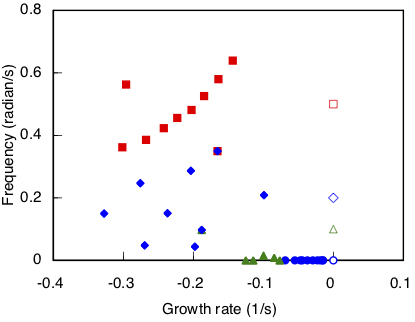In all this exploration we have not found the unstable complex pair of eigenvalues because we have used a shift to the left of this pair on the real axis. Repeating these tests with the real part of the shift set to 0.05, just slightly to the right of the known position of the leading eigenvalue, shows that an imaginary shift of 0.1i finds it, but the other values tested do not.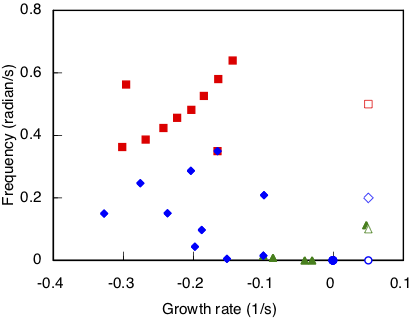The leading mode is a narrow target to find and I might have missed it altogether were it not for my discovery of a limit cycle during time integration of the nonlinear equations. And I missed that at first, too, until I started playing around with the time step as described in the "Breaking bad" entry below. This is somewhat disturbing, and it's a reminder of the hazards inherent to analyzing nonlinear equations.

Besides nonlinearity, what also makes this problem challenging to analyze is the large separation of time scales of the various physical phenomena that are involved. Interface motion occurs on a scale of hours, thermal transport occurs on a scale of minutes, and hydrodynamics occurs on a scale of seconds. A large number of eigenvalues that represent uninteresting modes are clustered near the origin, making it harder to find the unstable mode we seek.

This all reminds me of something.Ultimately understanding the eigenspectrum is about understanding the time scales of a problem. But it seems like we need to have a good understanding of those time scales before we study the eigenspectrum. Otherwise we're just guessing where to search the waters of the complex plane.

Wrap up

It doesn't take much for thermal convection in this system to become unstable. I suspect that time-dependent flow is commonplace in vertical Bridgman systems of industrial size. The impact might not always be significant, but certainly it has been documented that time-dependent flow can cause observable striations in some cases. Striations might purely be caused by time-dependent composition variations in the melt, but periodic melting back of the interface might also be involved. I expect to tackle this analysis soon.